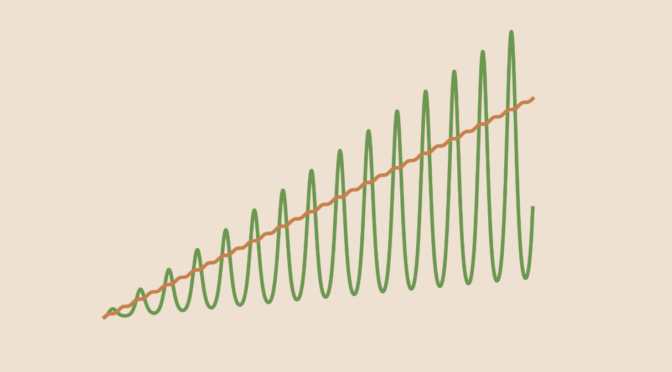# Counterexample around L’Hôpital’s rule

Let us consider two differentiable functions $$f$$ and $$g$$ defined in an open interval $$(a,b)$$, where $$b$$ might be $$\infty$$. If
$\lim\limits_{x \to b^-} f(x) = \lim\limits_{x \to b^-} g(x) = \infty$ and if $$g^\prime(x) \neq 0$$ in some interval $$(c,b)$$, then a version of l’Hôpital’s rule states that $$\lim\limits_{x \to b^-} \frac{f^\prime(x)}{g^\prime(x)} = L$$ implies $$\lim\limits_{x \to b^-} \frac{f(x)}{g(x)} = L$$.

We provide a counterexample when $$g^\prime$$ vanishes in all neighborhood of $$b$$. The counterexample is due to the Austrian mathematician Otto Stolz.

We take $$(0,\infty)$$ for the interval $$(a,b)$$ and $\begin{cases} f(x) &= x + \cos x \sin x\\ g(x) &= e^{\sin x}(x + \cos x \sin x) \end{cases}$ which derivatives are $\begin{cases} f^\prime(x) &= 2 \cos^2 x\\ g^\prime(x) &= e^{\sin x} \cos x (x + \cos x \sin x + 2 \cos x) \end{cases}$ We have $\lim\limits_{x \to \infty} \frac{f^\prime(x)}{g^\prime(x)} = \lim\limits_{x \to \infty} \frac{2 \cos x}{e^{\sin x} (x + \cos x \sin x + 2 \cos x)} = 0,$ however $\frac{f(x)}{g(x)} = \frac{1}{e^{\sin x}}$ doesn’t have any limit at $$\infty$$ as it oscillates between $$\frac{1}{e}$$ and $$e$$.